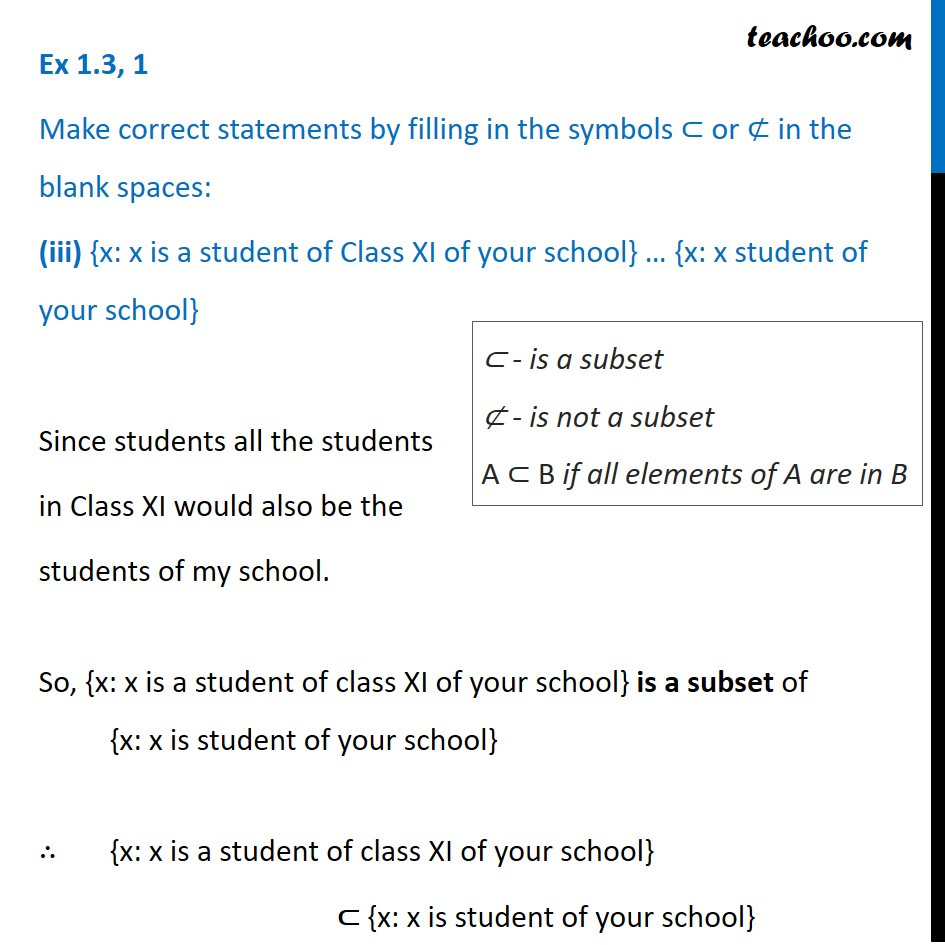1. Chapter 1 Class 11 Sets (Term 1)
2. Serial order wise
3. Ex 1.3

Transcript

Ex 1.3, 1 Make correct statements by filling in the symbols ⊂ or ⊄ in the blank spaces: (iii) {x: x is a student of Class XI of your school} … {x: x student of your school} Since students all the students in Class XI would also be the students of my school. So, {x: x is a student of class XI of your school} is a subset of {x: x is student of your school} ∴ {x: x is a student of class XI of your school} ⊂ {x: x is student of your school} ⊂ - is a subset ⊄ - is not a subset A ⊂ B if all elements of A are in B